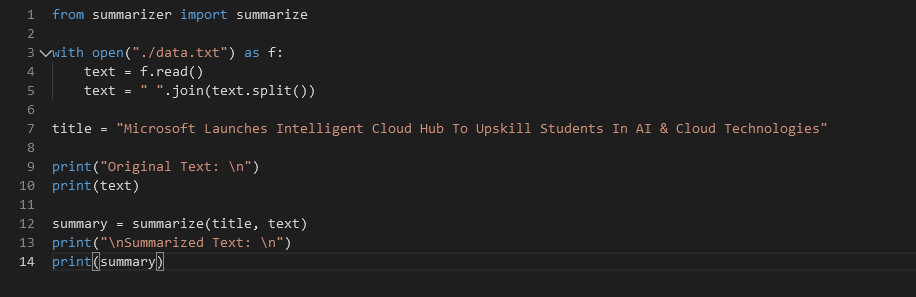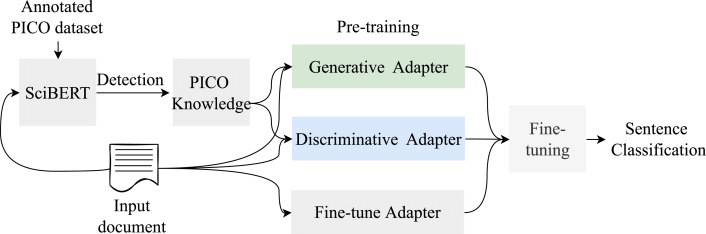# How to Summarize Text with Python and Deep Learning

Deep learning is one of the key essentials of AI. Expert predictions project the Deep Learning market to be worth around 21 billion by 2027. On the other hand, you have one of the most versatile development languages, Python.

Around 48% of developers use Python to create advanced-level applications. But how do these help with text summarization? Text summarization is one of the key essentials in many writing types. Academic writers need it, and so do marketers for various purposes.

One such example would be blogs having their tiny summaries in the shape of a social media caption. Another example would be a student writing a summary for their assignment/essay. Now, it’s important to understand that summarizing tools do not work magically.

They use intricate algorithms, and most of the good ones today employ Deep Learning and Python. So, how does it work, and how do you summarize text using Python and Deep Learning? Let’s find out.

## Understanding Text Summarization

Text summarization is the process of providing a brief yet detailed summary of the primary text. Compared to any primary body of text, summaries tend to be much shorter—usually around 10-20% of the entire text.

So, let’s say the primary text is around 2000 words, then the summaries tend to be within 200-300 words. There are a few purposes for summaries, such as:

• Providing a brief yet articulate view of the primary text;
• Building up the contents of the primary text by analyzing methodologies and research in it;
• Explaining the motivation and purpose behind creating a text—mostly relevant in academic texts;
• And to surmise the primary idea of any type of text.

Then it comes down to the various approaches to summarization. Manual summarization is just perusing the content and presenting a brief version of it in your own words.

Then people use various techniques to create summaries, such as abstractive and extractive summaries. Let’s keep digging to find out how these two summary types work.

## 4 Ways to Summarize Text with Python and Deep Learning

Now that the intricacies of summaries are out of the way let’s talk about the four ways Python and Deep Learning can be used for summarizing.

### Way 1: Extractive Summarization with Python

Extractive summarizing with Python is one of the leading ways to summarize any text with Python and deep learning techniques. It depends on three main parameters called Title, Text, and Count. Here’s what they mean:

• Title determines the main subject/title of your article/content;
• Text is the part where text-to-be summarized is used;
• And Count is the part that decides the length/sentences of the summary.

It is also imperative to focus on the Return value, as this parameter focuses on creating a summary based on the most important points in the text. Then comes the code in Python that summarizes, and it looks like this:It’s a 14-line code that summarizes any text. Here’s what each line does:

• Line-1 focuses on importing the summarizing method;
• Line-2-11 are self-explanatory;
• Line-12 is where we input summarized text;
• And Line-14 generates the summary.

This will churn out a basic summary of the text being input in Line-12.

### Way 2: Abstractive Summarization with Deep Learning

An abstractive summary is when the summarizing model extracts the idea and creates a summary in new words. Let’s say there are four sentences; then, an extractive summary would take sentences two and three to create a summary.

Whereas an abstractive summary would take ideas from all four sentences and write a new, smaller one. Abstractive summary through deep learning focuses on two encoder-decoder systems that look like this:This is the primary NLP model that’s used in text summarization. This sort of text summarization is possible with Python Libraries such as Numpy, Scipy, TensorFlow, etc.

### Way 3: Pre-trained Language Models for Summarization

Pre-trained language models for summarizations make writing a summary very easy. You can call these Python frameworks much like the one mentioned earlier in the article.

There are three main types of pre-trained models n Python that work for summarization, such as:

Their precursors are SciBERT and PICO Knowledge models. These two models extract the content from the input document and then pass it onward to one or more of the three pre-trained models. A visual representation of this system would look like this:So, if you were to use these models in Python-based paraphrasing, then it would look like this:

• Sentence classification layer;
• Inter-sentence transforming;
• And transformer encoder, i.e., the pre-trained model.

This method is a mixture of both extractive and subtractive summaries. However, it relies on existing content to formulate new sentences in a summary.

### Way 4: Using Python Libraries

Python offers powerful libraries that can handle this task with simplicity and efficiency. By incorporating these Python libraries into your workflow, you can save time and effort in summarizing text, making it a valuable tool for various applications; two such models in Python libraries are:

• NLTK is, another popular Python library is NLTK (Natural Language Toolkit). With NLTK, you can utilize algorithms and techniques to extract the most important information from a text and generate a concise summary.
• Gensim is the second option, which provides a straightforward way to perform text summarization. By leveraging algorithms like TextRank, Gensim can identify key phrases and sentences that capture the essence of the original text, allowing you to generate a condensed summary.

Using these libraries involves a few simple steps, such as tokenizing the text into sentences, removing stop words (common words like “the” or “and”), and calculating the relevance of each sentence. Once you have the scores, you can select the most relevant sentences to create a summary that captures the main points of the text.

Conclusion

These are some of the most efficient ways of summarizing text with Python and Deep Learning. It’s important to understand that text summarization is a process of various intricacies. It requires a bit of work to get things up and running with Python, Deep Learning, or Both. Hope that you will have understood how to summarize text with Python and deep learning.